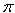SEARCH HOMEMath Central Quandaries & QueriesQuestion from Eilis, a student: How do I solve (1+i) to the power of 2-i? i.e. (1+i)^(2-i)Hi Eilis,

You need to use the exponential form to calculate this power. First of all

1 + i = √2 ei(/4 + 2n)               (1)

for any integer n. Also

1 + i = eln(1 + i)

Thus

(1 + i)2 - i = e (2 - i) ln(1 + i)

Thus using equation (1) the exponent is

(2 - i) ln(1 + i) = (2 - i) [ln(√2) + i(/4 + 2n)]

Expand this expression and simplify.

PennyMath Central is supported by the University of Regina and The Pacific Institute for the Mathematical Sciences.